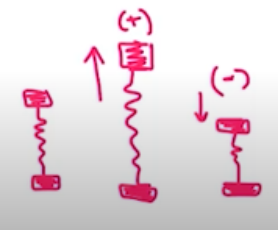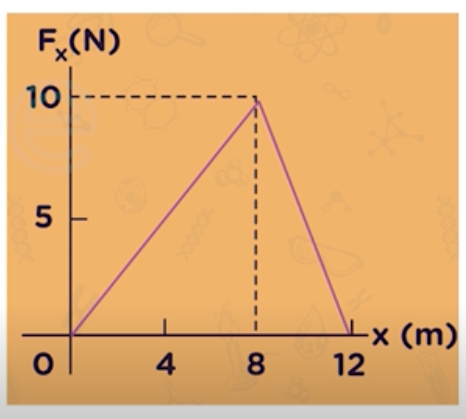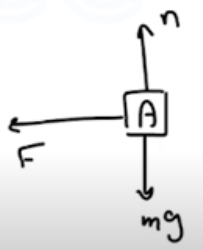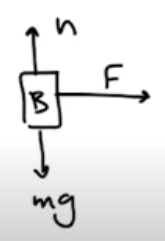Need Help?

Subscribe to Physics 1

###### \${selected_topic_name}
• Notes

$\begin{array}{l}{\text { To stretch a spring } 3.00 \mathrm{cm} \text { from its unstretched length, }} \\ {12.0 \mathrm{J} \text { of work must be done. (a) What is the force constant of }} \\ {\text { this spring? (b) What magnitude force is needed to stretch the }} \\ {\text { spring } 3.00 \mathrm{cm} \text { from its unstretched length? (c) How much work }} \\ {\text { must be done to compress this spring } 4.00 \mathrm{cm} \text { from its }} \\ {\text { unstretched length, and what force is needed to compress it this }} \\ {\text { distance? }}\end{array}$

$x_{1}=0 \quad, \quad w=\frac{1}{2} k{ x_{2}}^{2} \rightarrow k=\frac{2 w}{{x_{2}}^{2}}=\frac{2(12)}{(0.03)^{2}}=2.67 \times 10^{4} \mathrm{N/m}$

$F_{x}=K_ x=2.67 * 10^{4} * 0.03=801 N$$x_{1}=0, \quad x_{2}=-0.04 \mathrm{m}$

$w=\frac{1}{2}\left(2.67 * 10^{4}\right)(-0.04)^{2}=21.4 J$

$F_{x}=k x=\left(2.67 * 10^{4}\right)(0.04)=1070 \mathrm{N}$

$\begin{array}{l}{\text { A child applies a force } \vec{F} \text { parallel to the } x \text { -axis to a }} \\ {10.0 \text { -kg sled moving on the frozen surface of a small pond. }} \\ {\text { As the child controls the speed of the sled, the } x \text { -component of }} \\ {\text { the force she applies varies with the } x \text { -coordinate of the sled as }} \\ {\text { shown in Fig. E6.34. Calculate the work done by the force } \vec{F}} \\ {\text { when the sled moves (a) from } x=0 \text { to } x=8.0 \mathrm{m} ; \text { (b) from }} \\ {x=8.0 \mathrm{m} \text { to } x=12.0 \mathrm{m} ;(\mathrm{c}) \text { from } x=0 \text { to } 12.0 \mathrm{m} .}\end{array}$(a) $0 \longrightarrow 8$

$w=\frac{1}{2} * \text { base } * \text { height }=\frac{1}{2}(8)(10)=40 \mathrm{J}$

(b) $8 \rightarrow 12$

$w=\frac{1}{2} * \text { base } * \text { height }=\frac{1}{2}(4)(10)=20 J$

(c) $0 \rightarrow 12$

$w=\frac{1}{2} * \text { base* height }=\frac{1}{2}(12)(10)=60 \mathrm{J}$

$\begin{array}{l}{\text { A } 2.0-\mathrm{kg} \text { box and a } 3.0 \text { -kg box on a perfectly smooth hori- }} \\ {\text { zontal floor have a spring of force constant } 250 \mathrm{N} / \mathrm{m} \text { compressed }} \\ {\text { between them. If the initial compression of the spring is } 6.0 \mathrm{cm},} \\ {\text { find the acceleration of each box the instant after they are released. }} \\ {\text { Be sure to include free-body diagrams of each box as part of your }} \\ {\text { solution. }}\end{array}$$\sum F=m a$

$\rightarrow F=k|x|=250 * 0.06=15 N$

$a_{A}=\frac{\sum F}{m}=\frac{15}{2}=(7.5) \mathrm{m} / \mathrm{s}^{2}$

$a_{B}=\frac{\sum F}{m}=\frac{15}{3}=5 m / s^{2}$

$\begin{array}{l}{\text { A force in the }+x \text { -direction with magnitude }} \\ {F(x)=18.0 \mathrm{N}-(0.530 \mathrm{N} / \mathrm{m}) x \text { is applied to a } 6.00-\mathrm{kg} \text { box that }} \\ {\text { is sitting on the horizontal, frictionless surface of a frozen lake. }} \\ {F(x) \text { is the only horizontal force on the box. If the box is initially }} \\ {\text { at rest at } x=0, \text { what is its speed after it has traveled } 14.0 \mathrm{m} ?}\end{array}$

$w_{\text {total }}=\Delta k=k_{2}-k_{1}=\frac{1}{2} {m v_{2}}^{2}-\frac{1}{2} {m v_{1}}^{2}$

$w_{total}=\int_{x_{1}}^{x_{2}} F(x) d x$

$\rightarrow w_{\text {total }}=\int_{0}^{14}[18-(0.53) x] d x$

$=18(14)-(0.265)(14)^{2}=252 J-51.94 J-200.1 \mathrm{J}$

$w_{tot}=k_{2}=\frac{1}{2} m {v_{2}}^{2} \longrightarrow 200.1=\frac{1}{2}(6) {v_{2}}^{2}$

$∴ v_2=\sqrt{\frac{2(200.1)}{6}}=8.17 m/s$